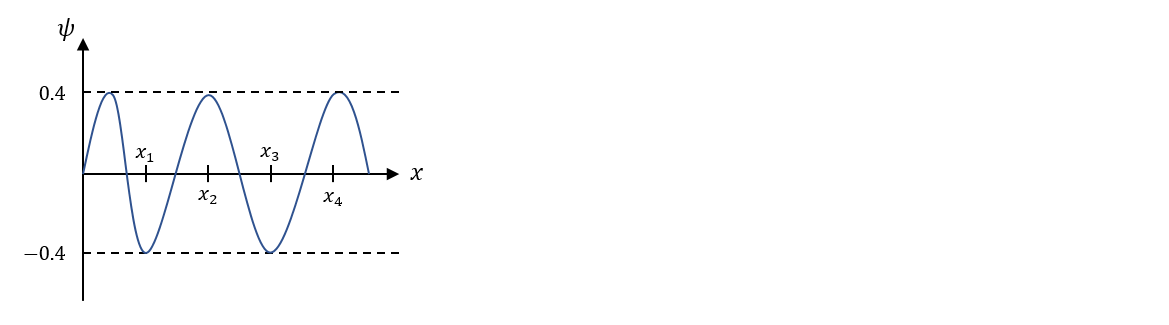# Problem: The wave function of a particle is shown in the figure below. What is the probability of finding the particle at x1? Assuming the wave function is symmetric, what is the probability of finding the particle at any position between 0 and x2?

###### Problem Details

The wave function of a particle is shown in the figure below. What is the probability of finding the particle at x1? Assuming the wave function is symmetric, what is the probability of finding the particle at any position between 0 and x2?